Introduction¶

This notebook is an implementation of Jón Daníelsson's Financial Risk Forecasting (Wiley, 2011) in Python 3.7.6, with annotations and introductory examples. The introductory examples (Appendix) are similar to Appendix B/C in the original book, with an emphasis on the differences between R/MATLAB and Python.

Bullet point numbers correspond to the R/MATLAB Listing numbers in the original book, referred to henceforth as FRF.

More details can be found at the book website: https://www.financialriskforecasting.com/

Last updated: July 2020

Copyright 2011-2020 Jón Daníelsson. This code is free software: you can redistribute it and/or modify it under the terms of the GNU General Public License as published by the Free Software Foundation, either version 3 of the License, or (at your option) any later version. This code is distributed in the hope that it will be useful, but WITHOUT ANY WARRANTY; without even the implied warranty of MERCHANTABILITY or FITNESS FOR A PARTICULAR PURPOSE. See the GNU General Public License for more details. The GNU General Public License is available at: https://www.gnu.org/licenses/.

Appendix: An Introduction to Python¶

Created in Python 3.7.6 (July 2020)

• P.1: Entering and Printing Data
• P.2: Vectors, Matrices and Sequences
• P.3: Importing Data (to be updated)
• P.4: Basic Summary Statistics
• P.5: Calculating Moments
• P.6: Basic Matrix Operations
• P.7: Statistical Distributions
• P.8: Statistical Tests
• P.9: Time Series
• P.10: Loops and Functions
• P.11: Basic Graphs
• P.12: Miscellaneous Useful Functions
In :
# Entering and Printing Data in Python
# Listing P.1
# Last updated June 2018
#
#

x = 10             # assign x the value 10
print(x)           # print the value of x
10
In :
# Vectors, Matrices and Sequences in Python
# Listing P.2
# Last updated July 2020
#
#

y = [1,3,5,7,9]       # lists in square brackets are stored as arrays

print(y)

print(y)           # 3rd element (Python indices start at 0)

print(len(y))         # as expected, y has length 5

import numpy as np    # NumPy: Numeric Python package

v = np.full([2,3], np.nan) # create a 2x3 matrix with NaN values

print(v)

print(v.shape)        # as expected, v is size (2,3)

w = np.tile([1,2,3], (3,2)) # repeats thrice by rows, twice by columns

print(w)

s = range(10)         # an iterator from 0 to 9

print([x for x in s]) # return  elements using list comprehension
[1, 3, 5, 7, 9]
5
5
[[nan nan nan]
[nan nan nan]]
(2, 3)
[[1 2 3 1 2 3]
[1 2 3 1 2 3]
[1 2 3 1 2 3]]
[0, 1, 2, 3, 4, 5, 6, 7, 8, 9]
In :
# Basic Summary Statistics in Python
# Listing P.3
# Last updated July 2020
#
#

import numpy as np

y = [3.14, 15, 9.26, 5]

print(sum(y))          # sum of all elements of y
print(max(y))          # maximum value of y
print(min(y))          # minimum value of y
print(np.mean(y))      # arithmetic mean
print(np.median(y))    # median
print(np.var(y))       # population variance
print(np.cov(y))       # covar matrix = sample variance for single vector
print(np.corrcoef(y))  # corr matrix =  for single vector
print(np.sort(y))      # sort in ascending order
print(np.log(y))       # natural log
32.4
15
3.14
8.1
7.13
20.791800000000002
27.7224
1.0
[ 3.14  5.    9.26 15.  ]
[1.1442228  2.7080502  2.22570405 1.60943791]
In :
# Calculating Moments in Python
# Listing P.4
# Last updated June 2018
#
#

from scipy import stats

print(np.mean(y))                        # mean
print(np.var(y))                         # variance
print(np.std(y, ddof = 1))               # ddof = 1 for unbiased standard deviation
print(stats.skew(y))                     # skewness
print(stats.kurtosis(y, fisher = False)) # fisher = False gives Pearson definition
8.1
20.791800000000002
5.265206548655049
0.47004939528995604
1.7153138938095185
In :
# Basic Matrix Operations in Python
# Listing P.5
# Last updated June 2018
#
#

z = np.matrix([[1, 2], [3, 4]])                   # z is a 2 x 2 matrix
x = np.matrix([1, 2])                             # x is a 1 x 2 matrix

## Note: z * x is undefined since the two matrices are not conformable

print(z * np.transpose(x))                        # this evaluates to a 2 x 1 matrix

b = np.concatenate((z,x), axis = 0)               # "stacking" z and x vertically

print(b)

c = np.concatenate((z,np.transpose(x)), axis = 1) # "stacking" z and x horizontally

print(c)

## note: dimensions must match along the combining axis
[[ 5]
]
[[1 2]
[3 4]
[1 2]]
[[1 2 1]
[3 4 2]]
In :
# Statistical Distributions in Python
# Listing P.6
# Last updated July 2020
#
#

q = np.arange(-3,4,1)        # specify a set of values, syntax arange(min, exclusive-max, step)

p = np.arange(0.1,1.0,0.1)   # specify a set of probabilities

print(stats.norm.ppf(p))     # element-wise inverse Normal quantile

print(stats.t.cdf(q,4))      # element-wise cdf under Student-t(4)

print(stats.chi2.pdf(q,2))   # element-wise pdf under Chisq(2)

## One can also obtain pseudorandom samples from distributions using numpy.random

x = np.random.standard_t(df=5, size=100)   # Sampling 100 times from TDist with 5 df

y = np.random.normal(size=50)              # Sampling 50 times from a standard normal

## Given data, we obtain MLE estimates of parameters with stats:

res = stats.norm.fit(x)                    # Fitting x to normal dist

print(res)                                 # First element is mean, second sd
[-1.28155157 -0.84162123 -0.52440051 -0.2533471   0.          0.2533471
0.52440051  0.84162123  1.28155157]
[0.01997098 0.05805826 0.18695048 0.5        0.81304952 0.94194174
0.98002902]
[0.         0.         0.         0.5        0.30326533 0.18393972
0.11156508]
(-0.031589776571727894, 1.3181920463588)
In :
# Statistical Tests in Python
# Listing P.7
# Last updated July 2020
#
#

from statsmodels.stats.diagnostic import acorr_ljungbox

x = np.random.standard_t(df=5, size=500)  # Create dataset x

print(stats.jarque_bera(x))               # Jarque-Bera test - prints statistic and p-value
print(acorr_ljungbox(x, lags=20))         # Ljung-Box test - prints array of statistics and p-values
(55.52193190760502, 8.781864124784988e-13)
(array([ 0.75917083,  0.76214466,  3.87993575,  3.88275209,  5.55279676,
7.42564775,  9.37830407,  9.37852858, 10.5152109 , 10.52877994,
12.2060809 , 12.58412229, 12.61226859, 14.61662675, 15.3165381 ,
16.22528148, 18.64862631, 18.98343826, 19.48099924, 19.55510145]), array([0.38358813, 0.68312848, 0.27472417, 0.42210612, 0.35219125,
0.28326929, 0.22662407, 0.31137647, 0.31040618, 0.39538755,
0.34835389, 0.39997837, 0.47818703, 0.40485275, 0.42886588,
0.43735143, 0.34906939, 0.39284402, 0.42639351, 0.48605098]))
In :
# Time Series in Python
# Listing P.8
# Last updated June 2018
#
#

import statsmodels.api as sm
import matplotlib.pyplot as plt

y = np.random.standard_t(df = 5, size = 60)   # Create hypothetical dataset y

q1 = sm.tsa.stattools.acf(y, nlags=20)        # autocorrelation for lags 1:20
plt.bar(x = np.arange(1,len(q1)), height = q1[1:])
plt.show()
plt.close()

q2 = sm.tsa.stattools.pacf(y, nlags=20)       # partial autocorr for lags 1:20
plt.bar(x = np.arange(1,len(q2)), height = q2[1:])
plt.show()
plt.close()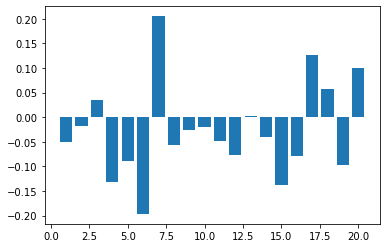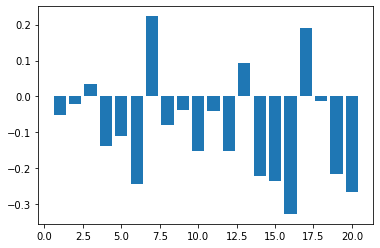In :
# Loops and Functions in Python
# Listing P.9
# Last updated June 2018
#
#

## For loops

for i in range(3,8):      # NOTE: range(start, end), end excluded
print(i**2)           # range(3,8) iterates through [3,4,5,6,7)

## If-else loops

X = 10

if X % 3 == 0:
print("X is a multiple of 3")
else:
print("X is not a multiple of 3")

## Functions (example: a simple excess kurtosis function)

def excess_kurtosis(x, excess = 3):        # note: excess optional, default = 3
m4=np.mean((x-np.mean(x))**4)          # note: exponentiation in Python uses **
excess_kurt=m4/(np.std(x)**4)-excess
return excess_kurt

x = np.random.standard_t(df=5,size=60)     # Create hypothetical dataset x

print(excess_kurtosis(x))
9
16
25
36
49
X is not a multiple of 3
1.4236668172208198
In :
# Basic Graphs in Python
# Listing P.10
# Last updated July 2020
#
#

y = np.random.normal(size = 50)
z = np.random.standard_t(df = 4, size = 50)

## using Matplotlib to plot bar, line, histogram and scatter plots
## subplot(a,b,c) creates a axb grid and plots the next plot in position c

plt.subplot(2,2,1)
plt.bar(range(len(y)), y);
plt.subplot(2,2,2)
plt.plot(y);
plt.subplot(2,2,3)
plt.hist(y);
plt.subplot(2,2,4)
plt.scatter(y,z);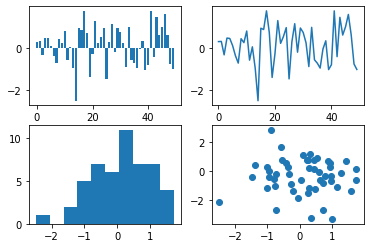In :
# Miscellaneous Useful Functions in Python
# Listing P.11
# Last updated June 2018
#
#

## Convert objects from one type to another with int(), float() etc
## To check type, use type(object)

x = 8.0

print(type(x))

x = int(x)

print(type(x))
<class 'float'>
<class 'int'>

Chapter 1: Financial Markets, Prices and Risk¶

• 1.3/1.4: Summary statistics for returns timeseries
• 1.5/1.6: Autocorrelation function (ACF) plots, Ljung-Box test
• 1.7/1.8: Quantile-Quantile (QQ) plots
• 1.9/1.10: Correlation matrix between different stocks
In :
# Listing 1.1/1.2
# Last updated July 2020
#
#

import numpy as np
import matplotlib.pyplot as plt

price = np.loadtxt('index.csv', delimiter = ',', skiprows = 1)

y = np.diff(np.log(price), n=1, axis=0)

plt.plot(y)
plt.title("S&P500 Returns")
plt.show()
plt.close()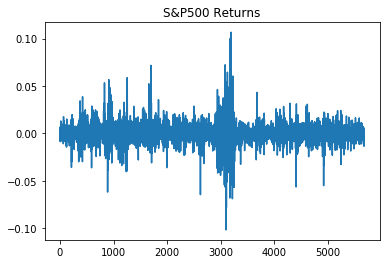In :
# Sample statistics in Python
# Listing 1.3/1.4
# Last updated July 2020
#
#

from scipy import stats

print (np.mean(y))
print (np.std(y, ddof=1))
print (np.min(y))
print (np.max(y))
print (stats.skew(y))
print (stats.kurtosis(y, fisher = False))
print (stats.jarque_bera(y))
0.00025815990082599837
0.010005728643763066
-0.10195548627302298
0.10673589502911707
0.1526332698963366
16.981171461092003
(46251.44013954825, 0.0)
In :
# ACF plots and the Ljung-Box test in Python
# Listing 1.5/1.6
# Last updated July 2020
#
#

import statsmodels.api as sm
import matplotlib.pyplot as plt
from statsmodels.stats.diagnostic import acorr_ljungbox

q = sm.tsa.stattools.acf(y, nlags=20)
plt.bar(x = np.arange(1,len(q)), height = q[1:])
plt.title("Autocorrelation of returns")
plt.show()
plt.close()

q = sm.tsa.stattools.acf(np.square(y), nlags=20)
plt.bar(x = np.arange(1,len(q)), height = q[1:])
plt.title("Autocorrelation of returns squared")
plt.show()
plt.close()

print (acorr_ljungbox(y, lags=20))
print (acorr_ljungbox(np.square(y), lags=20))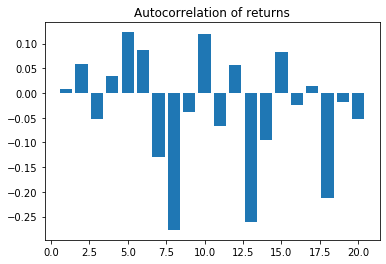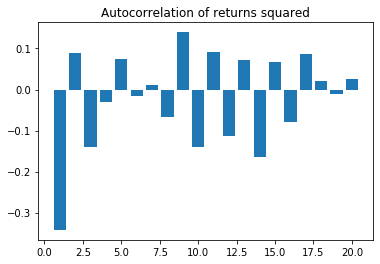(array([2.80924732e-03, 1.89460817e-01, 3.47105293e-01, 4.13990005e-01,
1.28820911e+00, 1.74523130e+00, 2.76966412e+00, 7.51652383e+00,
7.61355182e+00, 8.53457944e+00, 8.82978096e+00, 9.05287912e+00,
1.38422447e+01, 1.44873819e+01, 1.49944713e+01, 1.50415951e+01,
1.50598153e+01, 1.87323779e+01, 1.87582513e+01, 1.89918698e+01]), array([0.95773005, 0.90961813, 0.95093886, 0.98131556, 0.93614026,
0.94157619, 0.90546145, 0.48206107, 0.57350985, 0.57676746,
0.63760084, 0.69840734, 0.38504704, 0.41406729, 0.45181553,
0.52159416, 0.59117311, 0.40847456, 0.47244025, 0.52235455]))
(array([ 6.2177382 ,  6.65574735,  7.74579886,  7.79421128,  8.11615273,
8.13161875,  8.14033139,  8.42536996,  9.66126137, 10.93182139,
11.49625738, 12.38653765, 12.76172925, 14.71213587, 15.04959904,
15.53756963, 16.1381534 , 16.16964329, 16.179441  , 16.24044927]), array([0.01264766, 0.03586929, 0.05156806, 0.0994141 , 0.14994933,
0.22861834, 0.32038252, 0.39305881, 0.37859735, 0.36285439,
0.40267204, 0.41515662, 0.46638036, 0.39811133, 0.44785042,
0.48567367, 0.51406302, 0.58070915, 0.64527392, 0.70159958]))
In :
# QQ plots in Python
# Listing 1.7/1.8
# Last updated June 2018
#
#

from statsmodels.graphics.gofplots import qqplot

fig1 = qqplot(y, line='q', dist = stats.norm, fit = True)
plt.show()
plt.close()

fig2 = qqplot(y, line='q', dist = stats.t, distargs=(5,), fit = True)
plt.show()
plt.close()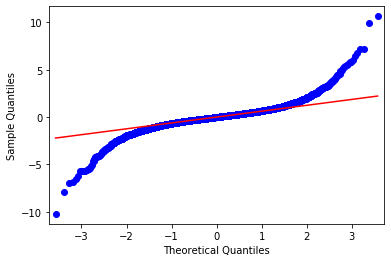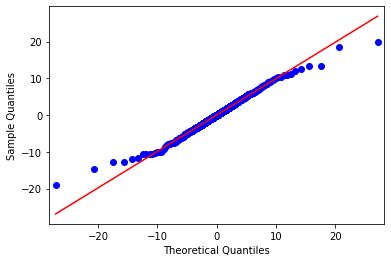In :
# Listing 1.9/1.10
# Last updated July 2020
#
#

y = np.diff(np.log(p), n=1, axis=0)

print(np.corrcoef(y, rowvar=False)) # correlation matrix

## rowvar=False indicates that columns are variables
[[1.         0.22968419 0.21261918]
[0.22968419 1.         0.14505109]
[0.21261918 0.14505109 1.        ]]

Chapter 2: Univariate Volatility Modelling¶

• 2.1/2.2: GARCH and t-GARCH estimation
• 2.3/2.4: APARCH estimation (unavailable as of June 2018)
In :
# START
# ARCH and GARCH estimation in Python
# Listing 2.1/2.2
# Last updated July 2020
#
#

import numpy as np

p = np.loadtxt('index.csv', delimiter = ',', skiprows = 1)

y = np.diff(np.log(p), n=1, axis=0)*100

y = y-np.mean(y)

from arch import arch_model
## using Kevin Sheppard's ARCH package for Python

## ARCH(1)
am = arch_model(y, mean = 'Zero', vol='Garch', p=1, o=0, q=0, dist='Normal')
arch1 = am.fit(update_freq=5, disp = "off")

## ARCH(4)
am = arch_model(y, mean = 'Zero', vol='Garch', p=4, o=0, q=0, dist='Normal')
arch4 = am.fit(update_freq=5, disp = "off")

## GARCH(4,1)
am = arch_model(y, mean = 'Zero', vol='Garch', p=4, o=0, q=1, dist='Normal')
garch4_1 = am.fit(update_freq=5, disp = "off")

## GARCH(1,1)
am = arch_model(y, mean = 'Zero', vol='Garch', p=1, o=0, q=1, dist='Normal')
garch1_1 = am.fit(update_freq=5, disp = "off")

## t-GARCH(1,1)
am = arch_model(y, mean = 'Zero', vol='Garch', p=1, o=0, q=1, dist='StudentsT')
tgarch1_1 = am.fit(update_freq=5, disp = "off")

print("ARCH(1) model:", "\n", arch1.summary(), "\n")
print("ARCH(4) model:", "\n", arch4.summary(), "\n")
print("GARCH(4,1) model:", "\n", garch4_1.summary(), "\n")
print("GARCH(1,1) model:", "\n", garch1_1.summary(), "\n")
print("tGARCH(1,1) model:", "\n", tgarch1_1.summary(), "\n")
ARCH(1) model:
Zero Mean - ARCH Model Results
==============================================================================
Dep. Variable:                      y   R-squared:                       0.000
Mean Model:                 Zero Mean   Adj. R-squared:                  0.000
Vol Model:                       ARCH   Log-Likelihood:               -7670.48
Distribution:                  Normal   AIC:                           15345.0
Method:            Maximum Likelihood   BIC:                           15358.3
No. Observations:                 5676
Date:                Wed, Jul 08 2020   Df Residuals:                     5674
Time:                        18:45:52   Df Model:                            2
Volatility Model
========================================================================
coef    std err          t      P>|t|  95.0% Conf. Int.
------------------------------------------------------------------------
omega          0.6846  4.051e-02     16.900  4.511e-64 [  0.605,  0.764]
alpha       0.3406  5.594e-02      6.088  1.143e-09 [  0.231,  0.450]
========================================================================

Covariance estimator: robust

ARCH(4) model:
Zero Mean - ARCH Model Results
==============================================================================
Dep. Variable:                      y   R-squared:                       0.000
Mean Model:                 Zero Mean   Adj. R-squared:                  0.000
Vol Model:                       ARCH   Log-Likelihood:               -7278.14
Distribution:                  Normal   AIC:                           14566.3
Method:            Maximum Likelihood   BIC:                           14599.5
No. Observations:                 5676
Date:                Wed, Jul 08 2020   Df Residuals:                     5671
Time:                        18:45:52   Df Model:                            5
Volatility Model
==========================================================================
coef    std err          t      P>|t|    95.0% Conf. Int.
--------------------------------------------------------------------------
omega          0.3892  3.601e-02     10.808  3.155e-27   [  0.319,  0.460]
alpha       0.1788  3.608e-02      4.955  7.249e-07   [  0.108,  0.249]
alpha       0.1539  3.143e-02      4.898  9.676e-07 [9.233e-02,  0.216]
alpha       0.1711  5.084e-02      3.366  7.640e-04 [7.146e-02,  0.271]
alpha       0.1432  3.428e-02      4.177  2.948e-05 [7.601e-02,  0.210]
==========================================================================

Covariance estimator: robust

GARCH(4,1) model:
Zero Mean - GARCH Model Results
==============================================================================
Dep. Variable:                      y   R-squared:                       0.000
Mean Model:                 Zero Mean   Adj. R-squared:                  0.000
Vol Model:                      GARCH   Log-Likelihood:               -6964.28
Distribution:                  Normal   AIC:                           13940.6
Method:            Maximum Likelihood   BIC:                           13980.4
No. Observations:                 5676
Date:                Wed, Jul 08 2020   Df Residuals:                     5670
Time:                        18:45:52   Df Model:                            6
Volatility Model
=============================================================================
coef    std err          t      P>|t|       95.0% Conf. Int.
-----------------------------------------------------------------------------
omega          0.0131  3.703e-03      3.539  4.010e-04  [5.848e-03,2.036e-02]
alpha       0.0460  1.697e-02      2.711  6.708e-03  [1.274e-02,7.925e-02]
alpha       0.0242  2.372e-02      1.019      0.308 [-2.231e-02,7.068e-02]
alpha       0.0000  2.998e-02      0.000      1.000 [-5.877e-02,5.877e-02]
alpha       0.0000  2.442e-02      0.000      1.000 [-4.787e-02,4.787e-02]
beta        0.9162  1.361e-02     67.333      0.000      [  0.890,  0.943]
=============================================================================

Covariance estimator: robust

GARCH(1,1) model:
Zero Mean - GARCH Model Results
==============================================================================
Dep. Variable:                      y   R-squared:                       0.000
Mean Model:                 Zero Mean   Adj. R-squared:                  0.000
Vol Model:                      GARCH   Log-Likelihood:               -6965.88
Distribution:                  Normal   AIC:                           13937.8
Method:            Maximum Likelihood   BIC:                           13957.7
No. Observations:                 5676
Date:                Wed, Jul 08 2020   Df Residuals:                     5673
Time:                        18:45:52   Df Model:                            3
Volatility Model
============================================================================
coef    std err          t      P>|t|      95.0% Conf. Int.
----------------------------------------------------------------------------
omega          0.0128  3.353e-03      3.803  1.427e-04 [6.181e-03,1.932e-02]
alpha       0.0668  8.769e-03      7.613  2.685e-14 [4.957e-02,8.394e-02]
beta        0.9198  1.115e-02     82.503      0.000     [  0.898,  0.942]
============================================================================

Covariance estimator: robust

tGARCH(1,1) model:
Zero Mean - GARCH Model Results
====================================================================================
Dep. Variable:                            y   R-squared:                       0.000
Mean Model:                       Zero Mean   Adj. R-squared:                  0.000
Vol Model:                            GARCH   Log-Likelihood:               -6551.58
Distribution:      Standardized Student's t   AIC:                           13111.2
Method:                  Maximum Likelihood   BIC:                           13137.7
No. Observations:                 5676
Date:                      Wed, Jul 08 2020   Df Residuals:                     5672
Time:                              18:45:52   Df Model:                            4
Volatility Model
============================================================================
coef    std err          t      P>|t|      95.0% Conf. Int.
----------------------------------------------------------------------------
omega          0.0175  3.107e-03      5.640  1.696e-08 [1.143e-02,2.361e-02]
alpha       0.0787  9.045e-03      8.702  3.264e-18 [6.098e-02,9.644e-02]
beta        0.9048  9.727e-03     93.016      0.000     [  0.886,  0.924]
Distribution
========================================================================
coef    std err          t      P>|t|  95.0% Conf. Int.
------------------------------------------------------------------------
nu             3.9448      0.210     18.752  1.862e-78 [  3.532,  4.357]
========================================================================

Covariance estimator: robust

In :
# Advanced ARCH and GARCH estimation in Python
# Listing 2.3/2.4
# Last updated July 2020
#
#

## Leverage effects
am = arch_model(y, mean = 'Zero', vol='Garch', p=1, o=1, q=1, dist='Normal')
leverage_garch1_1 = am.fit(update_freq=5, disp = "off")

## Power models, delta = 1
am = arch_model(y, mean = 'Zero', vol='Garch', p=1, o=0, q=1, dist='Normal', power = 1.0)
power_garch1_1 = am.fit(update_freq=5, disp = "off")

print("Leverage effects:", "\n", leverage_garch1_1.summary(), "\n")
print("Power model:", "\n", power_garch1_1.summary(), "\n")
Leverage effects:
Zero Mean - GJR-GARCH Model Results
==============================================================================
Dep. Variable:                      y   R-squared:                       0.000
Mean Model:                 Zero Mean   Adj. R-squared:                  0.000
Vol Model:                  GJR-GARCH   Log-Likelihood:               -6965.83
Distribution:                  Normal   AIC:                           13939.7
Method:            Maximum Likelihood   BIC:                           13966.2
No. Observations:                 5676
Date:                Wed, Jul 08 2020   Df Residuals:                     5672
Time:                        18:45:54   Df Model:                            4
Volatility Model
=============================================================================
coef    std err          t      P>|t|       95.0% Conf. Int.
-----------------------------------------------------------------------------
omega          0.0128  3.349e-03      3.813  1.371e-04  [6.207e-03,1.934e-02]
alpha       0.0656  1.207e-02      5.435  5.491e-08  [4.192e-02,8.922e-02]
gamma   2.4769e-03  1.632e-02      0.152      0.879 [-2.950e-02,3.446e-02]
beta        0.9197  1.108e-02     83.014      0.000      [  0.898,  0.941]
=============================================================================

Covariance estimator: robust

Power model:
Zero Mean - AVGARCH Model Results
==============================================================================
Dep. Variable:                      y   R-squared:                       0.000
Mean Model:                 Zero Mean   Adj. R-squared:                  0.000
Vol Model:                    AVGARCH   Log-Likelihood:               -7000.79
Distribution:                  Normal   AIC:                           14007.6
Method:            Maximum Likelihood   BIC:                           14027.5
No. Observations:                 5676
Date:                Wed, Jul 08 2020   Df Residuals:                     5673
Time:                        18:45:54   Df Model:                            3
Volatility Model
============================================================================
coef    std err          t      P>|t|      95.0% Conf. Int.
----------------------------------------------------------------------------
omega          0.0189  6.882e-03      2.741  6.127e-03 [5.374e-03,3.235e-02]
alpha       0.0795  1.137e-02      6.997  2.609e-12   [5.725e-02,  0.102]
beta        0.9205  1.579e-02     58.310      0.000     [  0.890,  0.951]
============================================================================

Covariance estimator: robust

Chapter 3: Multivariate Volatility Models¶

• 3.3/3.4: EWMA estimation
• 3.5/3.6: OGARCH estimation (unavailable as of June 2018)
• 3.7/3.8: DCC estimation (unavailable as of June 2018)
• 3.9/3.10: Comparison of EWMA, OGARCH, DCC (unavailable as of June 2018)
In :
# Listing 3.1/3.2
# Last updated June 2018
#
#

import numpy as np

p = p[:,[0,1]]                          # consider first two stocks

y = np.diff(np.log(p), n=1, axis=0)*100 # calculate returns

y[:,0] = y[:,0]-np.mean(y[:,0])         # subtract mean
y[:,1] = y[:,1]-np.mean(y[:,1])

T = len(y[:,0])
In :
# EWMA in Python
# Listing 3.3/3.4
# Last updated June 2018
#
#

EWMA = np.full([T,3], np.nan)

lmbda = 0.94

S = np.cov(y, rowvar = False)

EWMA[0,] = S.flatten()[[0,3,1]]

for i in range(1,T):
S = lmbda * S + (1-lmbda) * np.transpose(np.asmatrix(y[i-1]))* np.asmatrix(y[i-1])
EWMA[i,] = [S[0,0], S[1,1], S[0,1]]

EWMArho = np.divide(EWMA[:,2], np.sqrt(np.multiply(EWMA[:,0],EWMA[:,1])))

print(EWMArho)
[0.22968419 0.22363473 0.22182769 ... 0.34366321 0.31227409 0.31597027]
In :
# OGARCH in Python
# Listing 3.5/3.6
# Last updated July 2020
#
#

## Python does not have a proper OGARCH package at present
In :
# DCC in Python
# Listing 3.7/3.8
# Last updated July 2020
#
#

## Python does not have a proper DCC package at present
In :
# Correlation comparison in Python
# Listing 3.9/3.10
# Last updated July 2020
#
#

## Python does not have a proper OGARCH/DCC package at present

Chapter 4: Risk Measures¶

• 4.1/4.2: Expected Shortfall (ES) estimation under normality assumption
In :
# ES in Python
# Listing 4.1/4.2
# Last updated July 2020
#
#

from scipy import stats

p = [0.5, 0.1, 0.05, 0.025, 0.01, 0.001]
VaR = -stats.norm.ppf(p)
ES = stats.norm.pdf(stats.norm.ppf(p))/p

for i in range(len(p)):
print("VaR " + str(round(p[i]*100,3)) + "%: " + str(round(VaR[i],3)))
print("ES " + str(round(p[i]*100,3)) + "%: " + str(round(ES[i],3)), "\n")
VaR 50.0%: -0.0
ES 50.0%: 0.798

VaR 10.0%: 1.282
ES 10.0%: 1.755

VaR 5.0%: 1.645
ES 5.0%: 2.063

VaR 2.5%: 1.96
ES 2.5%: 2.338

VaR 1.0%: 2.326
ES 1.0%: 2.665

VaR 0.1%: 3.09
ES 0.1%: 3.367

Chapter 5: Implementing Risk Forecasts¶

• 5.3/5.4: Univariate HS Value at Risk (VaR)
• 5.5/5.6: Multivariate HS VaR
• 5.7/5.8: Univariate ES VaR
• 5.9/5.10: Normal VaR
• 5.11/5.12: Portfolio Normal VaR
• 5.13/5.14: Student-t VaR
• 5.15/5.16: Normal ES VaR
• 5.17/5.18: Direct Integration Normal ES VaR
• 5.19/5.20: MA Normal VaR
• 5.21/5.22: EWMA VaR
• 5.23/5.24: Two-asset EWMA VaR
• 5.25/5.26: GARCH(1,1) VaR
In :
# Listing 5.1/5.2
# Last updated July 2020
#
#

import numpy as np
from scipy import stats

p = p[:,[0,1]]      # consider two stocks
## convert prices to returns, and adjust length

y1 = np.diff(np.log(p[:,0]), n=1, axis=0)
y2 = np.diff(np.log(p[:,1]), n=1, axis=0)

y = np.stack([y1,y2], axis = 1)
T = len(y1)
value = 1000 # portfolio value
p = 0.01 # probability
In :
# Univariate HS in Python
# Listing 5.3/5.4
# Last updated July 2020
#
#

from math import ceil

ys = np.sort(y1) # sort returns
op = ceil(T*p)    # p percent smallest
VaR1 = -ys[op - 1] * value

print(VaR1)
17.498224747930724
In :
# Multivariate HS in Python
# Listing 5.5/5.6
# Last updated July 2020
#
#

w = [0.3, 0.7]               # vector of portfolio weights
yp = np.squeeze(np.matmul(y, w)) # portfolio returns
yps = np.sort(yp)
VaR2= -yps[op - 1] * value

print(VaR2)
18.726262569293546
In :
# Univariate ES in Python
# Listing 5.7/5.8
# Last updated June 2018
#
#

ES1 = -np.mean(ys[:op]) * value

print(ES1)
22.56338810381287
In :
# Normal VaR in Python
# Listing 5.9/5.10
# Last updated June 2018
#
#

sigma = np.std(y1, ddof=1) # estimate volatility
VaR3 = -sigma * stats.norm.ppf(p) * value

print(VaR3)
14.94957154354662
In :
# Portfolio normal VaR in Python
# Listing 5.11/5.12
# Last updated June 2018
#
#

## portfolio volatility
sigma = np.sqrt(np.mat(np.transpose(w))*np.mat(np.cov(y,rowvar=False))*np.mat(w))[0,0]
## Note: [0,0] is to pull the first element of the matrix out as a float
VaR4 = -sigma * stats.norm.ppf(p) * value

print(VaR4)
17.041085456523806
In :
# Student-t VaR in Python
# Listing 5.13/5.14
# Last updated June 2018
#
#

scy1 = y1 * 100         # scale the returns
res = stats.t.fit(scy1)

sigma = res/100      # rescale volatility
nu = res

VaR5 = -sigma*stats.t.ppf(p,nu)*value

print(VaR5)
17.123433115229002
In :
# Normal ES in Python
# Listing 5.15/5.16
# Last updated June 2018
#
#

sigma = np.std(y1, ddof=1)
ES2 = sigma * stats.norm.pdf(stats.norm.ppf(p)) / p * value

print(ES2)
17.127193705870486
In :
# Direct integration ES in Python
# Listing 5.17/5.18
# Last updated June 2018
#
#

VaR = -stats.norm.ppf(p)
integrand = lambda q: q * stats.norm.pdf(q)
ES = -sigma * quad(integrand, -np.inf, -VaR) / p * value

print(ES)
17.12719370586835
In :
# MA normal VaR in Python
# Listing 5.19/5.20
# Last updated June 2018
#
#

WE = 20
for t in range(T-5,T+1):
t1 = t-WE
window = y1[t1:t]      # estimation window
sigma = np.std(window, ddof=1)
VaR6 = -sigma*stats.norm.ppf(p)*value
print (VaR6)
16.05049679536141
16.149103169475975
18.854346063820373
18.882124798913356
16.230531751938656
16.169762107305665
In :
# EWMA VaR in Python
# Listing 5.21/5.22
# Last updated June 2018
#
#

lmbda = 0.94
s11 = np.var(y1[0:30], ddof = 1)     # initial variance

for t in range(1, T):
s11 = lmbda*s11 + (1-lmbda)*y1[t-1]**2

VaR7 = -np.sqrt(s11)*stats.norm.ppf(p)*value

print(VaR7)
16.753443875002283
In :
# Two-asset EWMA VaR in Python
# Listing 5.23/5.24
# Last updated July 2020
#
#

## s is the initial covariance
s = np.cov(y, rowvar = False)
for t in range(1,T):
s = lmbda*s+(1-lmbda)*np.transpose(np.asmatrix(y[t-1,:]))*np.asmatrix(y[t-1,:])

sigma = np.sqrt((np.transpose(w)*s*w))[0,0]
## Note: [0,0] is to pull the first element of the matrix out as a float

VaR8 = -sigma * stats.norm.ppf(p) * value

print(VaR8)
20.50363287336398
In :
# GARCH VaR in Python
# Listing 5.25/5.26
# Last updated July 2020
#
#

from arch import arch_model

am = arch_model(y1, mean = 'Zero', vol='Garch', p=1, o=0, q=1, dist='Normal', rescale = False)
res = am.fit(update_freq=5, disp = "off")
omega = res.params.loc['omega']
alpha = res.params.loc['alpha']
beta = res.params.loc['beta']
## computing sigma2 for t+1
sigma2 = omega + alpha*y1[T-1]**2 + beta * res.conditional_volatility[-1]**2

VaR9 = -np.sqrt(sigma2) * stats.norm.ppf(p) * value

print(VaR9)
16.542817446347694

Chapter 6: Analytical Value-at-Risk for Options and Bonds¶

• 6.1/6.2: Black-Scholes function definition
• 6.3/6.4: Black-Scholes option price calculation example
In :
# Black-Scholes function in Python
# Listing 6.1/6.2
# Last updated June 2018
#
#

import numpy as np
from scipy import stats

def bs(X, P, r, sigma, T):
d1 = (np.log(P/X) + (r + 0.5 * sigma**2)*T)/(sigma * np.sqrt(T))
d2 = d1 - sigma * np.sqrt(T)

Call = P * stats.norm.cdf(d1) - X * np.exp(-r * T) * stats.norm.cdf(d2)
Put = X * np.exp(-r * T) * stats.norm.cdf(-d2) - P * stats.norm.cdf(-d1)

Delta_Call = stats.norm.cdf(d1)
Delta_Put = Delta_Call - 1
Gamma = stats.norm.pdf(d1) / (P * sigma * np.sqrt(T))

return {"Call": Call, "Put": Put, "Delta_Call": Delta_Call, "Delta_Put": Delta_Put, "Gamma": Gamma}
In :
# Black-Scholes in Python
# Listing 6.3/6.4
# Last updated July 2020
#
#

f = bs(X = 90, P = 100, r = 0.05, sigma = 0.2, T = 0.5)

print(f)
{'Call': 13.498517482637212, 'Put': 1.2764095651871656, 'Delta_Call': 0.8395228492806657, 'Delta_Put': -0.16047715071933433, 'Gamma': 0.017238257785615545}

Chapter 7: Simulation Methods for VaR for Options and Bonds¶

• 7.1/7.2: Plotting normal distribution transformation
• 7.3/7.4: Random number generation from Uniform(0,1), Normal(0,1)
• 7.5/7.6: Bond pricing using yield curve
• 7.7/7.8: Yield curve simulations
• 7.9/7.10: Bond price simulations
• 7.11/7.12: Black-Scholes analytical pricing of call
• 7.13/7.14: Black-Scholes Monte Carlo simulation pricing of call
• 7.15/7.16: Option density plots
• 7.17/7.18: VaR simulation of portfolio with only underlying
• 7.19/7.20: VaR simulation of portfolio with only call
• 7.21/7.22: VaR simulation of portfolio with call, put and underlying
• 7.23/7.24: Simulated two-asset returns
• 7.25/7.26: Two-asset portfolio VaR
• 7.27/7.28: Two-asset portfolio VaR with a call
In :
# Transformation in Python
# Listing 7.1/7.2
# Last updated July 2020
#
#

import numpy as np
import matplotlib.pyplot as plt

x = np.arange(-3,3.1, step = 0.1) # Python's arange excludes the last value
plt.plot(x, stats.norm.cdf(x))
plt.title("CDF of Normal distribution")
plt.show()
plt.close()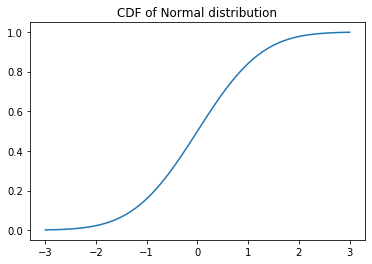In :
# Various RNs in Python
# Listing 7.3/7.4
# Last updated July 2020
#
#

np.random.seed(12)     # set seed
S = 10                 # simulation size

print (np.random.uniform(size = S))
print (np.random.normal(size = S))
print (np.random.standard_t(df = 4,size = S))
[0.15416284 0.7400497  0.26331502 0.53373939 0.01457496 0.91874701
0.90071485 0.03342143 0.95694934 0.13720932]
[ 0.75314283 -1.53472134  0.00512708 -0.12022767 -0.80698188  2.87181939
-0.59782292  0.47245699  1.09595612 -1.2151688 ]
[ 1.54289086  0.69652904  0.52148112 -0.25218158 -4.66442107 -2.42642183
0.27546574 -2.17082333  1.29967088  1.7842616 ]
In :
# Price bond in Python
# Listing 7.5/7.6
# Last updated July 2020
#
#

yield_c = [5.00, 5.69, 6.09, 6.38, 6.61,
6.79, 6.94, 7.07, 7.19, 7.30]    # yield curve

T = len(yield_c)
r = 0.07                                    # initial yield rate
Par = 10                                    # par value
coupon = r * Par                            # coupon payments
cc = [coupon] * 10                          # vector of cash flows
cc[T-1] += Par                              # add par to cash flows
P=np.sum(cc/(np.power((1+np.divide(yield_c,100)),
list(range(1,T+1))))) # calc price

print(P)
9.913205732444842
In :
# Simulate yields in Python
# Listing 7.7/7.8
# Last updated July 2020
#
#

np.random.seed(12)                   # set seed
sigma = 1.5                          # daily yield volatility
S = 8                                # number of simulations
r = np.random.normal(0,sigma,size=S) # generate random numbers

ysim = np.zeros([T,S])
for i in range(S):
ysim[:,i] = yield_c + r[i]

plt.plot(ysim)
plt.title("Simulated yield curves")
plt.show()
plt.close()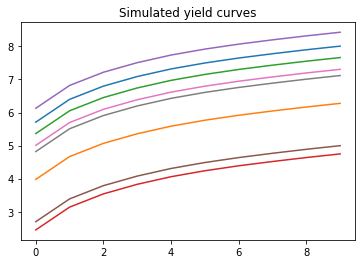In :
# Simulate bond prices in Python
# Listing 7.9/7.10
# Last updated November 2020
#
#

S = 8                   # simulation size
SP = np.zeros([S])      # initializing vector with zeros

for i in range(S):      # S simulations
SP[i] = np.sum(cc/(np.power((1+ysim[:,i]/100), list(range(1,T+1)))))

SP -= (np.mean(SP) - P) # correct for mean

plt.bar(range(1,S+1), SP)
plt.title("Simulated bond prices, S = 8")
plt.show()
plt.close()

S = 50000

np.random.seed(12)
r = np.random.normal(0, sigma, size = S)

ysim = np.zeros([T,S])
for i in range(S):
ysim[:,i] = yield_c + r[i]

SP = np.zeros([S])

for i in range(S):
SP[i] = np.sum(cc/(np.power((1+ysim[:,i]/100), list(range(1,T+1)))))

SP -= (np.mean(SP) - P)

plt.hist(SP, bins = 30, range = (7, 13), density = True)

fitted_norm=stats.norm.pdf(np.linspace(7,13,30),
np.mean(SP),np.std(SP,ddof=1))

plt.plot(np.linspace(7,13,30), fitted_norm)
plt.title("Histogram for simulated bond prices, S = 50000")
plt.show()
plt.close()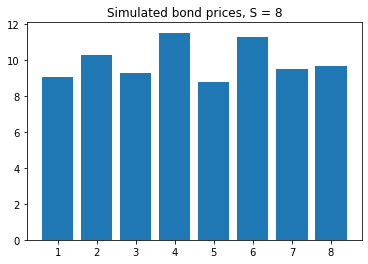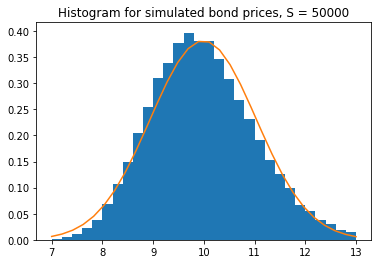In :
# Black-Scholes valuation in Python
# Listing 7.11/7.12
# Last updated July 2020
#
#

## This calculation uses the Black-Scholes pricing function (Listing 6.1/6.2)
P0 = 50                     # initial spot price
sigma = 0.2                 # annual volatility
r = 0.05                    # annual interest
T = 0.5                     # time to expiration
X = 40                      # strike price
f = bs(X, P0, r, sigma, T)  # analytical call price

print(f)
{'Call': 11.087280700718757, 'Put': 0.09967718185206187, 'Delta_Call': 0.9660259272621473, 'Delta_Put': -0.03397407273785269, 'Gamma': 0.010663779610020534}
In :
# Black-Scholes simulation in Python
# Listing 7.13/7.14
# Last updated July 2020
#
#

np.random.seed(12)          # set seed
S = 10**6                   # number of simulations
ysim=np.random.normal(-0.5*sigma**2*T,
sigma*np.sqrt(T),size=S) # sim returns, lognorm corrected
F = P0 * np.exp(r * T) * np.exp(ysim)          # sim future prices
SP = F - X                  # payoff
SP[SP < 0] = 0              # set negative outcomes to zero
fsim = SP * np.exp(-r * T)  # discount
call_sim = np.mean(fsim)    # simulated price
print(call_sim)
11.068936888001415
In :
# Option density plots in Python
# Listing 7.15/7.16
# Last updated July 2020
#
#

plt.hist(F, bins = 60, range = (20,80), density = True)
fitted_norm=stats.norm.pdf(np.linspace(20,80,60),np.mean(F),np.std(F,ddof=1))
plt.plot(np.linspace(20,80,60), fitted_norm)
plt.axvline(x=X, color='k')
plt.title("Density of future prices")
plt.show()
plt.close()

plt.hist(fsim, bins = 60, range = (0, 35), density = True)
plt.axvline(x=f['Call'], color='k')
plt.title("Density of Call option prices")
plt.show()
plt.close()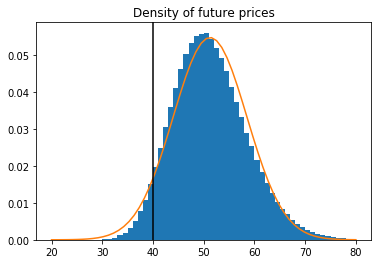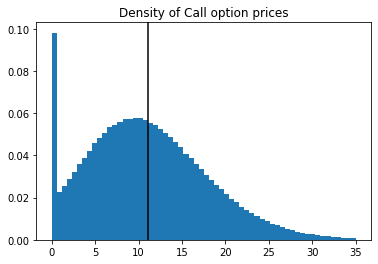In :
# Simulate VaR in Python
# Listing 7.17/7.18
# Last updated July 2020
#
#

from math import ceil

np.random.seed(1)       # set seed
S = 10**7               # number of simulations
s2 = 0.01**2            # daily variance
p = 0.01                # probability
r = 0.05                # annual riskfree rate
P = 100                 # price today
ysim=np.random.normal(r/365-0.5*s2,np.sqrt(s2),size=S) # sim returns
Psim = P * np.exp(ysim) # sim future prices
q = np.sort(Psim - P)   # simulated P/L
VaR1 = -q[ceil(p*S) - 1]

print(VaR1)
2.290784802622909
In :
# Simulate option VaR in Python
# Listing 7.19/7.20
# Last updated July 2020
#
#

T = 0.25       # time to expiration
X = 100                                 # strike price
sigma = np.sqrt(s2 * 250)               # annual volatility
f = bs(X, P, r, sigma, T)               # analytical call price
fsim = bs(X, Psim, r, sigma, T-(1/365)) # sim option prices
q = np.sort(fsim['Call']-f['Call'])     # simulated P/L
VaR2 = -q[ceil(p*S) - 1]

print(VaR2)
1.2150728853608541
In :
# Example 7.3 in Python
# Listing 7.21/7.22
# Last updated July 2020
#
#

X1 = 100
X2 = 110
f1 = bs(X1, P, r, sigma, T)
f2 = bs(X2, P, r, sigma, T)
f2sim = bs(X2, Psim, r, sigma, T-(1/365))
f1sim = bs(X1, Psim, r, sigma, T-(1/365))
q = np.sort(f1sim['Call'] + f2sim['Put'] + Psim - f1['Call'] - f2['Put'] - P)
VaR3 = -q[ceil(p*S) - 1]

print(VaR3)
1.4952375118324568
In :
# Simulated two-asset returns in Python
# Listing 7.23/7.24
# Last updated June 2018
#
#

np.random.seed(12)                                      # set seed
mu = np.transpose([r/365, r/365])                       # return mean
Sigma = np.matrix([[0.01, 0.0005],[0.0005, 0.02]])      # covariance matrix
y = np.random.multivariate_normal(mu, Sigma, size = S)  # simulated returns
In :
# Two-asset VaR in Python
# Listing 7.25/7.26
# Last updated July 2020
#
#

import numpy.matlib

P = np.asarray([100, 50])              # prices
x = np.asarray([1, 1])                 # number of assets
Port = np.matmul(P, x)                 # portfolio at t
Psim = np.matlib.repmat(P,S,1)*np.exp(y) # simulated prices
PortSim = np.matmul(Psim, x)           # simulated portfolio value
q = np.sort(PortSim - Port)            # simulated P/L
VaR4 = -q[ceil(p*S) - 1]

print(VaR4)
25.942795557394135
In :
# A two-asset case in Python with an option
# Listing 7.27/7.28
# Last updated July 2020
#
#

f = bs(X = P, P = P, r = r, sigma = sigma, T = T)
fsim = bs(X = P, P = Psim[:,1], r = r, sigma = sigma, T = T-(1/365))
q = np.sort(fsim['Call'] + Psim[:,0] - f['Call'] - P)
VaR5 = -q[ceil(p*S) - 1]

print(VaR5)
20.777617782200394

Chapter 8: Backtesting and Stress Testing¶

• 8.3/8.4: Setting up backtest
• 8.5/8.6: Running backtest for EWMA/MA/HS/GARCH VaR
• 8.7/8.8: Backtesting analysis for EWMA/MA/HS/GARCH VaR
• 8.9/8.10: Bernoulli coverage test
• 8.11/8.12: Independence test
• 8.13/8.14: Running Bernoulli/Independence test on backtests
• 8.15/8.16: Running backtest for EWMA/HS ES
• 8.17/8.18: Backtesting analysis for EWMA/HS ES
In :
# Listing 8.1/8.2
# Last updated June 2018
#
#

import numpy as np

y = np.diff(np.log(price), n=1, axis=0) # get returns
In :
# Set backtest up in Python
# Listing 8.3/8.4
# Last updated July 2020
#
#

from math import ceil

T = len(y)                   # number of obs for y
WE = 1000                    # estimation window length
p = 0.01                     # probability
l1 = ceil(WE * p)             # HS observation
value = 1                    # portfolio value
VaR = np.full([T,4], np.nan) # matrix for forecasts

## EWMA setup

lmbda = 0.94
s11 = np.var(y)

for t in range(1,WE):
s11=lmbda*s11+(1-lmbda)*y[t-1]**2
In :
# Running backtest in Python
# Listing 8.5/8.6
# Last updated July 2020
#
#

from scipy import stats
from arch import arch_model

for t in range(WE, T):
t1 = t - WE           # start of data window
t2 = t - 1            # end of data window
window = y[t1:t2+1]   # data for estimation

s11 = lmbda * s11 + (1-lmbda) * y[t-1]**2
VaR[t,0] = -stats.norm.ppf(p)*np.sqrt(s11)*value # EWMA

VaR[t,1] = -np.std(window,ddof=1)*stats.norm.ppf(p)*value # MA

ys = np.sort(window)
VaR[t,2] = -ys[l1 - 1] * value # HS

am = arch_model(window, mean = 'Zero',vol = 'Garch',
p = 1, o = 0, q = 1, dist = 'Normal', rescale = False)
res = am.fit(update_freq=0, disp = 'off', show_warning=False)
par = [res.params, res.params, res.params]
s4 = par + par * window[WE - 1]**2 + par[
2] * res.conditional_volatility[-1]**2
VaR[t,3] = -np.sqrt(s4) * stats.norm.ppf(p) * value # GARCH(1,1)
In :
# Backtesting analysis in Python
# Listing 8.7/8.8
# Last updated July 2020
#
#

W1 = WE # Python index starts at 0
m = ["EWMA", "MA", "HS", "GARCH"]

for i in range(4):
VR = sum(y[W1:T] < -VaR[W1:T,i])/(p*(T-WE))
s = np.std(VaR[W1:T, i], ddof=1)
print (m[i], "\n",
"Violation ratio:", round(VR, 3), "\n",
"Volatility:", round(s,3), "\n")

plt.plot(y[W1:T])
plt.plot(VaR[W1:T])
plt.title("Backtesting")
plt.show()
plt.close()
EWMA
Violation ratio: 2.074
Volatility: 0.012

MA
Violation ratio: 1.861
Volatility: 0.007

HS
Violation ratio: 1.24
Volatility: 0.012

GARCH
Violation ratio: 1.561
Volatility: 0.01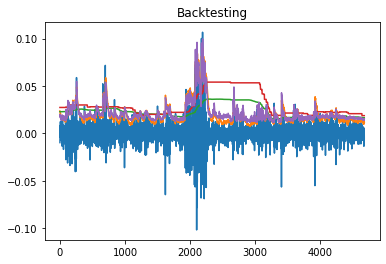In :
# Bernoulli coverage test in Python
# Listing 8.9/8.10
# Last updated June 2018
#
#

def bern_test(p,v):
lv = len(v)
sv = sum(v)

al = np.log(p)*sv + np.log(1-p)*(lv-sv)
bl = np.log(sv/lv)*sv + np.log(1-sv/lv)*(lv-sv)

return (-2*(al-bl))
In :
# Independence test in Python
# Listing 8.11/8.12
# Last updated June 2018
#
#

def ind_test(V):
J = np.full([T,4], 0)
for i in range(1,len(V)-1):
J[i,0] = (V[i-1] == 0) & (V[i] == 0)
J[i,1] = (V[i-1] == 0) & (V[i] == 1)
J[i,2] = (V[i-1] == 1) & (V[i] == 0)
J[i,3] = (V[i-1] == 1) & (V[i] == 1)

V_00 = sum(J[:,0])
V_01 = sum(J[:,1])
V_10 = sum(J[:,2])
V_11 = sum(J[:,3])

p_00=V_00/(V_00+V_01)
p_01=V_01/(V_00+V_01)
p_10=V_10/(V_10+V_11)
p_11=V_11/(V_10+V_11)

hat_p = (V_01+V_11)/(V_00+V_01+V_10+V_11)
al = np.log(1-hat_p)*(V_00+V_10) + np.log(hat_p)*(V_01+V_11)
bl = np.log(p_00)*V_00 + np.log(p_01)*V_01 + np.log(p_10)*V_10 + np.log(p_11)*V_11

return (-2*(al-bl))
In :
# Backtesting S&P 500 in Python
# Listing 8.13/8.14
# Last updated July 2020
#
#

W1 = WE
ya = y[W1:T]
VaRa = VaR[W1:T,]
m = ['EWMA', 'MA', 'HS', 'GARCH']

for i in range(4):
q = y[W1:T] < -VaR[W1:T,i]
v = VaRa*0
v[q,i] = 1
ber = bern_test(p, v[:,i])
ind = ind_test(v[:,i])
print (m[i], "\n",
"Bernoulli:", "Test statistic =", round(ber,3), "p-value =", round(1 - stats.chi2.cdf(ber, 1),3), "\n",
"Independence:", "Test statistic =", round(ind,3), "p-value =", round(1 - stats.chi2.cdf(ind, 1),3), "\n")
EWMA
Bernoulli: Test statistic = 41.626 p-value = 0.0
Independence: Test statistic = 0.441 p-value = 0.507

MA
Bernoulli: Test statistic = 27.904 p-value = 0.0
Independence: Test statistic = 17.439 p-value = 0.0

HS
Bernoulli: Test statistic = 2.535 p-value = 0.111
Independence: Test statistic = 11.483 p-value = 0.001

GARCH
Bernoulli: Test statistic = 12.702 p-value = 0.0
Independence: Test statistic = 0.549 p-value = 0.459

In :
# Backtest ES in Python
# Listing 8.15/8.16
# Last updated July 2020
#
#

VaR = np.full([T,2], np.nan) # VaR forecasts
ES = np.full([T,2], np.nan)  # ES forecasts
for t in range(WE, T):
t1 = t - WE
t2 = t - 1
window = y[t1:t2+1]

s11 = lmbda * s11 + (1-lmbda) * y[t-1]**2
VaR[t,0] = -stats.norm.ppf(p) * np.sqrt(s11)*value       # EWMA
ES[t,0]=np.sqrt(s11)*stats.norm.pdf(stats.norm.ppf(p))/p

ys = np.sort(window)
VaR[t,1] = -ys[l1 - 1] * value                           # HS
ES[t,1] = -np.mean(ys[0:l1]) * value
In :
# ES in Python
# Listing 8.17/8.18
# Last updated July 2020
#
#

ESa = ES[W1:T,:]
VaRa = VaR[W1:T,:]
m = ["EWMA", "HS"]

for i in range(2):
q = ya <= -VaRa[:,i]
nES = np.mean(ya[q] / -ESa[q,i])
print (m[i], 'nES = ', round(nES,3))
EWMA nES =  1.224
HS nES =  1.054

Chapter 9: Extreme Value Theory¶

• 9.1/9.2: Calculation of tail index from returns
In :
# Hill estimator in Python
# Listing 9.1/9.2
# Last updated June 2018
#
#

ysort = np.sort(y)                                # sort the returns
CT = 100                                          # set the threshold
iota = 1/(np.mean(np.log(ysort[0:CT]/ysort[CT]))) # get the tail index

print(iota)

# END
2.62970984651441